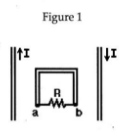# Problem: In Figure 1, two parallel wires carry a current I in opposite directions. A rectangular loop is midway between the wires. The current I is decreasing. The induced current through the resistor R is:  A) from b to a B) from a to b C) zero

🤓 Based on our data, we think this question is relevant for Professor Batzill's class at USF.

###### Problem Details

In Figure 1, two parallel wires carry a current I in opposite directions. A rectangular loop is midway between the wires. The current I is decreasing. The induced current through the resistor R is:

A) from b to a

B) from a to b

C) zero